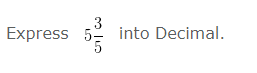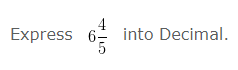# Convert Mixed Fraction to Decimal

Convert Mixed Fraction to Decimal – Example 1Solution

Whenever, we have a mixed fraction, it would consist of two parts.

Whole Number Part = 5

Fraction Part = 3/5

The Whole Number Part would remain the same.

The Fraction Part should be converted into decimal

When we convert 3/5 into decimal we would get 0.6

The Decimal value of the given fraction = Whole Number Part . Decimal value of Fraction Part

The Decimal value of the given fraction = 5.6

Convert Mixed Fraction to Decimal – Example 2Solution

Whenever, we have a mixed fraction, it would consist of two parts.

Whole Number Part = 6

Fraction Part = 4/5

The Whole Number Part would remain the same.

The Fraction Part should be converted into decimal

When we convert 4/5 into decimal we would get 0.8

The Decimal value of the given fraction = Whole Number Part . Decimal value of Fraction Part

The Decimal value of the given fraction = 6.8